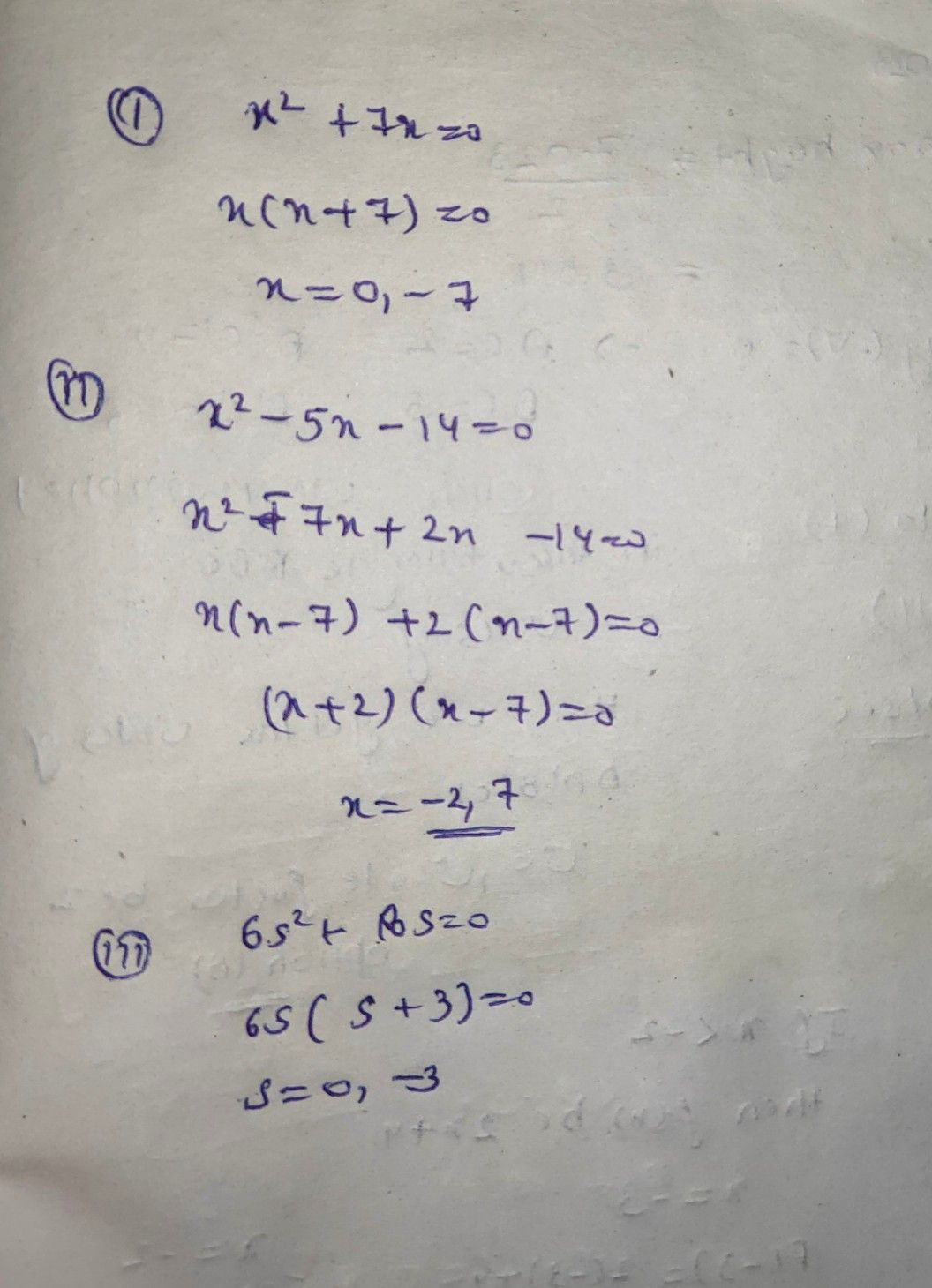Symbol
Problem$1$ Activity $4s$ Factor Then Solve! Solve the following quadratic equations by factoring. Answer the questions that follow. $1.$ $x^{2}+7x=0$ 6. $x^{2}-14=5x$ 2. $6s^{2}+18s=0$ $7$ $11r+15=-2r^{2}$ 3. $t^{2}+8t+16=0$ $8.$ $x^{2}-25=0$ $4$ $x^{2}-10x+25=0$ 9. $81-4x^{2}=0$ 5. $h^{2}+6h=16$ $10$ $4s^{2}+9=12s$ $Quesions:$ a. How did you find the solutions of each equation? b. What mathematics concepts or principles did you apply in finding the solutions? how you applied these. c. Compare your answers with those of your classmates. Did you arrive at the same s If NOT, explain.
7th-9th grade
Other
Search count: 126
SolutionQanda teacher - AskhinaThanks for asking a question. You can ask any doubt without any hesitation in a direct way also for high-quality answers quickly and please rate 5 stars if you are satisfied with my answers And, if you have no doubt related to the previous question then please evaluate the answer and give 3000 coins as a gift ( these are not reduced to your wallet)